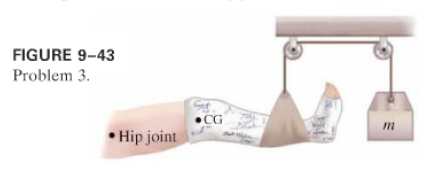×
Get Full Access to Physics: Principles With Applications - 6 Edition - Chapter 9 - Problem 3p
Get Full Access to Physics: Principles With Applications - 6 Edition - Chapter 9 - Problem 3p

×

# Calculate the mass m needed in order to suspend the legISBN: 9780130606204 3

## Solution for problem 3P Chapter 9

Physics: Principles with Applications | 6th Edition

• Textbook Solutions
• 2901 Step-by-step solutions solved by professors and subject experts
• Get 24/7 help from StudySoup virtual teaching assistantsPhysics: Principles with Applications | 6th Edition

4 5 1 256 Reviews
12
3
Problem 3P

(I) Calculate the massneeded in order to suspend the leg shown in Fig.Assume the leg (with cast) has a mass of, and itsisfrom the hip joint; the sling isfrom the hip joint.Step-by-Step Solution:

Step 1 0f 3 impulse is the measure of how much the force changes the momentum of an object. Impulse= force ×time= F × t p And we know that F = t So Impulse is J = p J =p fp i p =m(v) mfv) i

Step 2 of 3

Step 3 of 3

##### ISBN: 9780130606204

Unlock Textbook Solution